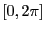Next: Example Up: Bound on the roots Previous: Bound on the roots   Contents

Implementation

This procedure return angle intervals included in the rangewhich contain the roots of the trigonometric equation.

int Bound_Root_Trigo_Interval(int n,VECTOR &A,INTEGER_VECTOR &SSin,
INTEGER_VECTOR &CCos,int *nbsol,VECTOR &Inf,VECTOR &Sup);
with:
• n: number of terms of the equation
• A: the coefficients of each term which may be either real or integer
• Ssin: the sine power of each term
• Ccos: the cosine power of each term
• nbsol: the number of angle intervals which are returned by the procedure (at most 4)
• Inf: the lower bound of the returned angle interval
• Sup: the upper bound of the returned angle interval
Note that the size of Inf, Sup should be 4 at least. In case of failure the procedure return -1, 1 on success.

Subsections

Jean-Pierre Merlet 2012-12-20